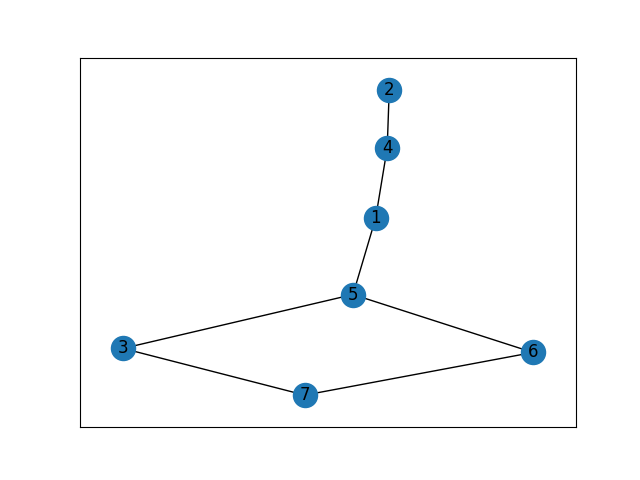•Gurobi Staff

Hi Yunzhuang,

This is expected behaviour in column generation.
In your case, it may happen that using the colourings already produced, all the vertices are covered and the entering column (even though it has a negative reduced cost), will not enter the basis in the current iteration.
It may become basic in combination with other future columns or not at all.
This results in the objective remaining the same for several iterations and then jumping.
This is expected, and as long as you have formulated the master (in your case: set covering) and pricing (maximum-weight independent set, MWIS), and you terminate when a column has a non-negative reduced cost you should be alright (in the case of the MWIS, the weight is > 1).

Cheers,
David

•Hi David,

Thanks for your quick explanation.

I cannot understand "it may happen that using the colorings already produced, all the vertices are covered and the entering column (even though it has a negative reduced cost), will not enter the basis in the current iteration.".

Existing columns covering all vertices make a feasible restricted master and produce some dual solution, but why is this preventing a new negative-reduced-cost column from entering the basis and making improvements?

Thanks, Yunzhuang

•Gurobi Staff

Hi Yunzhuang,

This happens because the duals for some of the nodes are non-zero. This is just because the gap between the primal and dual has not been closed. Having a column not entering the basis happens regularly as it may not be beneficial.

I've attached a rough implementation of Mehrotra and Trick (1996). This very small trivial example exhibits this behaviour.Iter 0 obj=7000.0        duals={4: 1000.0, 5: 1000.0, 6: 1000.0, 7: 1000.0, 1: 1000.0, 2: 1000.0, 3: 1000.0}        coloring=[6, 1, 2, 3]Iter 1 obj=3001.0        duals={4: 1000.0, 5: 1000.0, 6: 1.0, 7: 1000.0, 1: 0.0, 2: 0.0, 3: 0.0}        coloring=[4, 5, 7]Iter 2 obj=2.0        duals={4: 1.0, 5: 0.0, 6: 1.0, 7: 0.0, 1: 0.0, 2: 0.0, 3: 0.0}        coloring=[4, 6]Iter 3 obj=2.0        duals={4: 1.0, 5: 0.0, 6: 0.0, 7: 0.0, 1: 1.0, 2: 0.0, 3: 0.0}Solution found with 2 colorscolor: 0 nodes: [6, 1, 2, 3]color: 1 nodes: [4, 5, 7]

At iteration 1, even though node 6 is already in the restricted master, the dual remains non-zero until the very last iteration.
At iteration 2, the same thing happens with node 4 which is now also in the restricted master.
At iteration 3, either node 4 or node 1 could be in the coloring but this meets the termination criteria.

Clearly, we already have the optimal coloring at iteration 2, so any column produced after that will not enter the basis even though it has a non-negative reduced cost (>1 in this case) and is present in the restricted master.

Cheers,
David

---

Mehrotra, A., & Trick, M. A. (1996). A column generation approach for graph coloring. informs Journal on Computing, 8(4), 344-354.

---

import reimport networkx as nxfrom gurobipy import *# import matplotlib.pyplot as pltPENALTY = 1000def solve(G):    master, constrs = init_master(G)    itr = 0    wisd = {}    while True:        master.optimize()        print(f"Iter {itr} obj={master.objVal}")        duals = {k: v.Pi for k, v in constrs.items()}        print(f"\t{duals=}")        cost, wis = sub(duals, G, itr)        if cost > 1:            master = update_master(master, constrs, wis, itr)            wisd[itr] = wis            print(f"\tcoloring={wis}")        else:            break        itr += 1    varss = master.getVars()    colors = [get_int(v.VarName) for v in varss if v.X > 0]    print(f"Solution found with {len(colors)} colors")    for c in colors:        print(f"color: {c} nodes: {wisd[c]}")def init_master(G):    m = Model("Master problem (set covering)")    m.params.outputflag = 0    x = m.addVars(G.nodes(), lb=0.0, ub=GRB.INFINITY, name="x_dummy", obj=PENALTY)    constrs = m.addConstrs((x[n] >= 1 for n in G.nodes()), "V")    return m, constrsdef get_int(string: str):    return int(re.search(r"\d+", string).group())def update_master(master, constrs, wis, itr):    c = [v for k, v in constrs.items() if k in wis]    master.addVar(obj=1.0, column=Column([1.0] * len(wis), c), name=f"x_{itr}")    return masterdef sub(duals, G, itr):    m = Model(f"Subproblem {itr} (weighted independent set)")    m.params.outputflag = 0    z = m.addVars(G.nodes(), name="z", vtype=GRB.BINARY)    m.addConstrs((z[i] + z[j] <= 1 for (i, j) in G.edges()))    m.setObjective(sum(v * z[k] for k, v in duals.items()), GRB.MAXIMIZE)    m.optimize()    m.write(f"sub_{itr}.lp")    return m.objVal, [v for v in G.nodes() if z[v].X > 0]if __name__ == "__main__":    G = nx.Graph()    G.add_nodes_from([4, 5, 6, 7])    G.add_edge(1, 4)    G.add_edge(1, 5)    G.add_edge(2, 4)    G.add_edge(3, 5)    G.add_edge(5, 6)    G.add_edge(7, 3)    G.add_edge(7, 6)    # nx.draw_networkx(G, with_labels=True)    # plt.show()    solve(G)